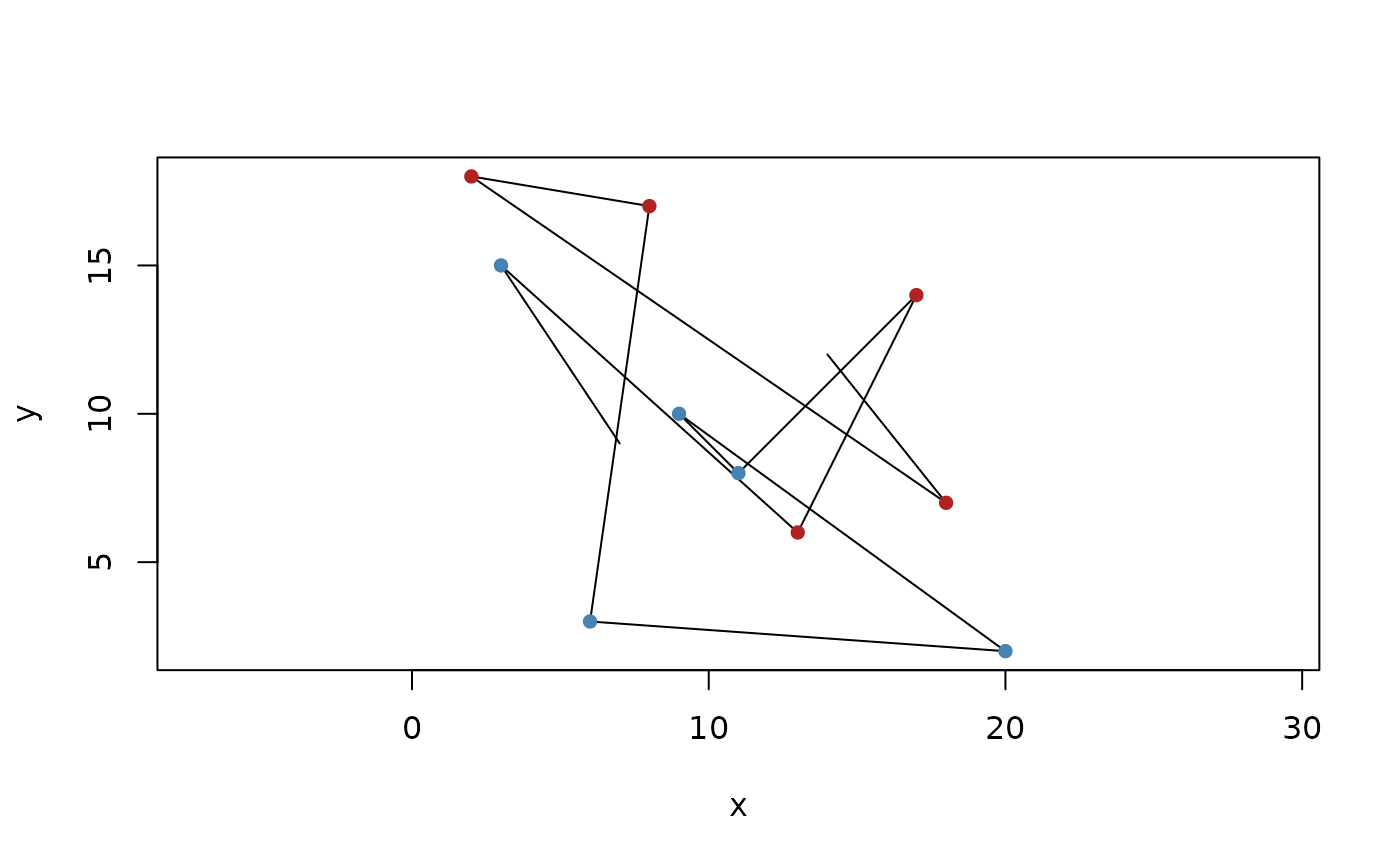In 2D the bend three consecutive points form can be oriented either to the left or two the right. Asking whether it takes a left turn is equivalent to asking whether z lies on the positive side of the supporting line of x->y. euclid provides two predicate functions to check whether left or right turns are constructed from three given point vectors (note that collinear points will be FALSE for both predicates). It also provides a function that calculate turn orientation along a single vector of points. The result of this are given as: 1 (left), 0 (collinear), or -1 (right). Note that the output of turn_along() is two elements shorter than the input.

turns_left(x, y, z)

turns_right(x, y, z)

turn_along(x)

## Arguments

x, y, z

Vectors of 2D points

## Value

Either a logical vector or an integer vector (for turn_along())

Other Predicates: collinear(), constant_in, geometry_class, has_intersection(), in_order(), is_degenerate(), location_predicates, parallel()

## Examples

p <- point(sample(20, 12), sample(20, 12))

turns_left(p[1:4], p[5:8], p[9:12])
#>   TRUE FALSE FALSE  TRUE

turn_along(p)
#>   -1 -1  1 -1  1  1  1  1  1  1

plot(segment(p[-12], p[-1]))
euclid_plot(p[-c(1, 12)][turn_along(p) == 1], pch = 16, col = "firebrick")
euclid_plot(p[-c(1, 12)][turn_along(p) == -1], pch = 16, col = "steelblue")